# Quiz 1: Business Decisions and Financial Accounting

1. Prepare a forecasted income statement for the year ended December 31, 2013: Income statement: Income statement measures company's financial performance for one accounting cycle. In this statement, total expenses are subtracted from total revenues. This income statement shows net income or loss for a particular period. An Income statement shows the results of the operations of a business for a period. It includes revenue and expense accounts and reports either a net income or a net loss. 1. The Revenue of the business is recorded first with dollar sign 2. Expenses are recorded next according to the order of chart of accounts. Only revenue and expenses balances are taken in income statement. 3. The total of the expenses is subtracted from the total revenue. If revenues exceed total expenses then it is net income. If total expenses exceed total revenue, the result is net loss.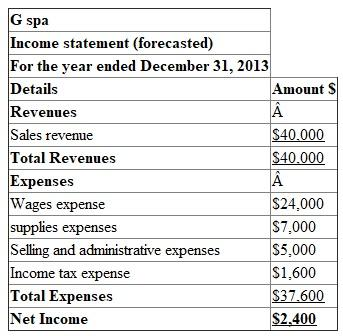2. Prepare a statement of Retained earnings for the year ended December 31, 2013: Beginning retained earnings plus net income minus dividends equals to retained earnings ending balance.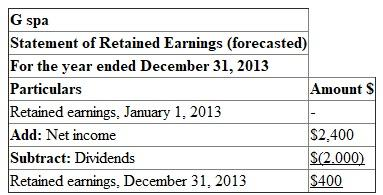3. Prepare a Balance sheet at December 31, 2013: The basic accounting equation is as follows: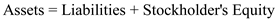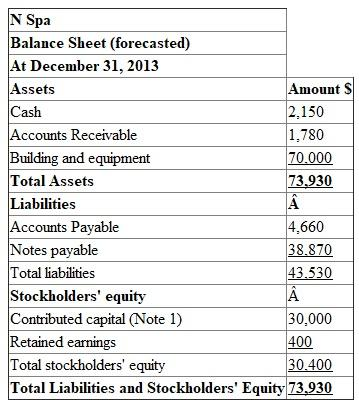Note 1: 3 people invested \$10,000 each where total contribution valued \$ 30,000. 4. As of December 31, 2013 most of the financing come from creditors but not from stockholders. As it is a first year of operations to N spa, there were no retained earnings at the beginning of the year. Hence, creditors' contribution is more in the current situation. Total contribution from creditors and stockholders are \$43,530 and \$30,400 respectively.
1. Income Statement: The first financial statement prepared is the income statement. The income statement reports the net amount that a business earned (net income) over a period of time by subtracting the costs of running the business (expenses) from the total amount earned (revenues).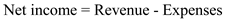Prepare an income statement for the year ended December 31, 2012: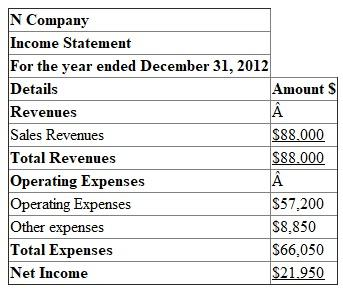2. Prepare a statement of Retained earnings for the year ended December 31, 2012: The statement of retained earnings explains changes in the Retained Earnings account over a period of time by considering increases (from net income) and decreases (from dividends to stockholders). Beginning retained earnings plus net income minus dividends equals to retained earnings ending balance.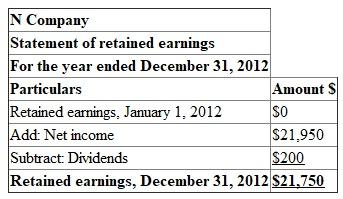3. Balance sheet's purpose is to report the amount of a business's assets, liabilities, and stockholders' equity at a specific point in time. Prepare a Balance sheet at December 31, 2012: The basic accounting equation is as follows: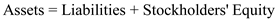Resources owned by the company called assets. Resources owed to creditors, and to stockholders are called liabilities and stockholders' equity.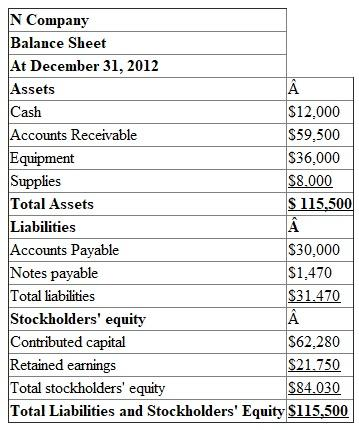(a). Calculate the balance sheet is in balance with equation: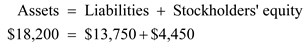(b) Calculate the net Income by using equation: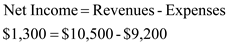(c) Calculate the changes in retained earnings by using equation: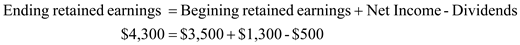(d) Calculate the changes in cash account by using equation: